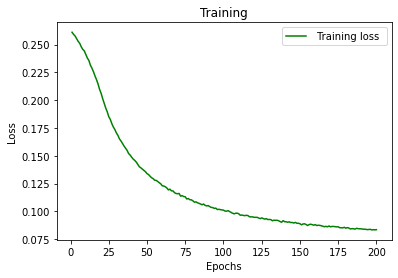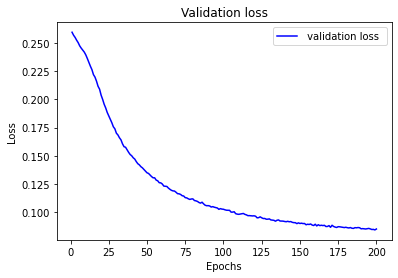# Adding Validation Loss to the model

Hi everyone.
For my project , i’m trying to predict the ratings that a user will give to an unseen movie, based on the ratings he gave to other movies. I’m using the movielens dataset.The Main folder, which is ml-100k contains informations about 100 000 movies.To create the recommendation systems, the model ‘Autoencoder’ is being used. I’m using Google Colab for coding implementation.

So far I managed to get the training loss values and plot them on the graph but I’m struggling to add the validation loss for this project.

Here’s my code.

r_cols = [‘user_id’, ‘movie_id’, ‘rating’, ‘timestamp’] #Create each column

user_ratings = pd.read_csv(‘u.data’, sep=‘\t’, names=r_cols, header = None, engine = ‘python’, encoding = ‘latin-1’) #Read the file

#Preparing Training Set and Test Set
train_data, test_data = train_test_split(user_ratings , test_size=0.20, random_state=42)

#Converting the training and test sets into numpy arrays
train_data = np.array(train_data, dtype = ‘int’)
test_data = np.array(test_data, dtype = ‘int’)

#We’ll need the number of users and the number of movies to build our recommendation system. Since id_users and id_movies start at index 1, the number of users represent the maximum value of id_user. Similarly, the number of movies represent the maximum value of id_movie. However, since the data is divided into training and test set, the maximum value of id_user/id_movie is either in the training_set or in the test_set.

#Getting the number of users and movies
nb_users = int(max(max(train_data[:, 0]), max(train_data[:, 0])))
nb_movies = int(max(max(test_data[:, 1]), max(test_data[:, 1])))

print(“Number of users: {}”.format(nb_users))
print(“Number of movies: {}”.format(nb_movies))

#Number of users: 943
#Number of movies: 1682

#In order to build the autoencoder, we need a specific data structure. In our case, we will create a list of lists, expected by Pytorch. Each list of list will contain the ratings that a specific user gave to the movies. If a user didn’t rate a movie, we’ll just add a 0 for that observation. We will define a function which will create this list of list for us.

def convert(data):
# Initializing an empty list that will take the list of ratings given by a specific user
new_data =
# Looping over all the users
for id_users in range(1, nb_users + 1):
# We get the id of the movies rated by the current user
id_movies = data[:, 1][data[:, 0] == id_users]
# We get the id of the ratings given by the current_user
id_ratings = data[:, 2][data[:, 0] == id_users]
ratings = np.zeros(nb_movies)
# For movies rated by the current user, we replace 0 with the rating
# The first element of ratings is at index 0. However, id_movies start at index 1.
# Therefore, ratings[id_movies - 1] will correspond to the location of the movie we’re considering
ratings[id_movies - 1] = id_ratings
new_data.append(list(ratings))
return new_data

#Applying the convert function to the training and test set.
train_data = convert(train_data)
test_data = convert(test_data)

#Convert the data into Torch tensors
train_data = torch.FloatTensor(train_data)
test_data = torch.FloatTensor(test_data)

batch_size = 200

‘’’ Dataset Class’‘’

class DatasetR(Dataset):

``````def __init__(self, train_data, nb_users, transform=None):

super(DatasetR, self).__init__()

self.train_data = train_data

self.nb_users = nb_users

def __len__(self):

return self.nb_users

def __getitem__(self, idx):

#print(idx)

sample = self.train_data[idx]

#sample = torch.Tensor(sample)

return sample
``````

#Creating the architecture of the Neural Network We’ll create a stacked autoencoder. This stacked autoencoder will get one input layer, two encoding layers and two decoding layers. As a reminder, for an autoencoder, the number of nodes of the output layer should equal the number of nodes of the input layer.

class SAE(nn.Module):

``````#Initializing the class

def __init__(self, ):

# making the class get all the functions from the parent class nn.Module

super(SAE, self).__init__()

# Creating the first encoding layer. The number of input corresponds to the number of movies

#  Decide to encode it into 20 outputs

self.fc1 = nn.Linear(nb_movies, 20)

# Creating the second encoding layer. From 20 inputs to 10 outputs

self.fc2 = nn.Linear(20, 10)

# Creating the first decoding layer. From 10 inputs to 20 outputs

self.fc3 = nn.Linear(10, 20)

# Creating the second hidden layer. From 20 inputs to nb_movies outputs

self.fc4 = nn.Linear(20, nb_movies)

# Creating the activation fucntion which will fire up specific neurons

self.activation = nn.Sigmoid()

# Creating the function for forward propagation

def forward(self, x):

# x = self.do1(self.bn1(self.activation(self.fc1(x))))

# x = self.do2(self.bn2(self.activation(self.fc2(x))))

# x = self.do3(self.bn3(self.activation(self.fc3(x))))

x = self.activation(self.fc1(x))

x = self.activation(self.fc2(x))

x = self.activation(self.fc3(x))

# With autoencoder, we don't need an activation function for the last decoding part

x = self.fc4(x)

return x
``````

#Creating an instance of our SAE class

sae = SAE()

dataset = DatasetR(train_data = train_data, nb_users = nb_users)

datasetTest = DatasetR(train_data = test_data, nb_users = nb_users)

criterion = nn.MSELoss()

#Defining the algorithm used to minimize the loss function. In this case, we’ll use RMSprop

optimizer = optim.RMSprop(sae.parameters(), lr = 0.01, weight_decay = 0.5)

#Setting the number of epochs

losses =

nb_epoch = 200

#Iterating over each epoch

for epoch in range(1, nb_epoch + 1):

``````#sae.train()

# Initializing the train_loss which will be updated

train_loss = 0

# Initializing a counter

s = 0.

#Iterating over each user

#for id_user in range(nb_users):

# The input corresponds to the ratings given by the current user for each movie

input = Variable(sample)

target = input.clone()

# We don't consider movies NOT rated by the current user. So we specify a conditional statement

if torch.sum(target.data > 0) > 0:

# We use our SAE to get the output from the

#print('input:  '+ str(input.shape))

output = sae(input)

#print(output.shape)

output[target == 0] = 0

# Defining our loss function, comparing the output with the target

loss = criterion(output, target)

mean_corrector = nb_movies/float(torch.sum(target.data > 0) + 1e-10)

loss.backward()

# Updating the train_loss

train_loss += np.sqrt(loss.data*mean_corrector)

s += 1.

# Updating the weights of the neural network

optimizer.step()

losses.append(epoch_loss)

print('epoch: '+str(epoch)+' loss: '+str(train_loss/s))
``````

plt.plot(losses)

plt.xlabel("Number of Epoch ")

plt.ylabel(" Training Loss")

plt.title(“Autoencoder : Loss vs Number of Epoch”)

plt.show()

After a training of 200 epochs, we get an overall lost of 0.0837. This means that for the training_set, we have : predicted_rating - 0.0837<= real_rating <= predicted_rating + 0.0837

Now I’m stuck on implementing the validation loss part.
Can anyone help me with this?
Thanks

You could reuse the training loop and just remove the `backward` and `optimizer.step()` calls.
Also, you could wrap the validation/test loop into a `with torch.no_grad()` block to save some memory.

Also note that `Variables` are deprecated since PyTorch `0.4` and you can use tensors now.

Hi @ptrblck
I modified the code below

``````# Setting the number of epochs

losses = []

tlosses = []

nb_epoch = 200

# Iterating over each epoch

for epoch in range(1, nb_epoch + 1):

#sae.train()

# Initializing the train_loss which will be updated

train_loss = 0

test_loss = 0

# Initializing a counter

s = 0.

# Iterating over each user

#for id_user in range(nb_users):

# The input corresponds to the ratings given by the current user for each movie

input = Variable(sample)

target = input.clone()

# We don't consider movies NOT rated by the current user. So we specify a conditional statement

if torch.sum(target.data > 0) > 0:

# We use our SAE to get the output from the

#print('input:  '+ str(input.shape))

output = sae(input)

#print(output.shape)

output[target == 0] = 0

# Defining our loss function, comparing the output with the target

loss = criterion(output, target)

mean_corrector = nb_movies/float(torch.sum(target.data > 0) + 1e-10)

loss.backward()

# Updating the train_loss

train_loss += np.sqrt(loss.data*mean_corrector)

s += 1.

# Updating the weights of the neural network

optimizer.step()

# The input corresponds to the ratings given by the current user for each movie

input = Variable(sample)

target = input.clone()

# We don't consider movies NOT rated by the current user. So we specify a conditional statement

if torch.sum(target.data > 0) > 0:

# We use our SAE to get the output from the

#print('input:  '+ str(input.shape))

output = sae(input)

#print(output.shape)

output[target == 0] = 0

# Defining our loss function, comparing the output with the target

loss = criterion(output, target)

mean_corrector = nb_movies/float(torch.sum(target.data > 0) + 1e-10)

# Updating the train_loss

test_loss += np.sqrt(loss.data*mean_corrector)

s += 1.

losses.append(epoch_loss)

tlosses.append(epoch_loss1)

print('epoch: '+str(epoch)+' Training loss: '+str(train_loss/s))

print('epoch: '+str(epoch)+' Test loss: '+str(test_loss/s))

``````

When I plot the training loss and Validation loss graphs .They look basically the same.

``````epoch: 1 Training loss: tensor(0.1306)
epoch: 1 Test loss: tensor(0.1298)
epoch: 2 Training loss: tensor(0.1296)
epoch: 2 Test loss: tensor(0.1285)
epoch: 3 Training loss: tensor(0.1289)
epoch: 3 Test loss: tensor(0.1275)
epoch: 4 Training loss: tensor(0.1277)
epoch: 4 Test loss: tensor(0.1263)
epoch: 5 Training loss: tensor(0.1265)
epoch: 5 Test loss: tensor(0.1252)
.........
.......
.......
epoch: 195 Training loss: tensor(0.0419)
epoch: 195 Test loss: tensor(0.0428)
epoch: 196 Training loss: tensor(0.0420)
epoch: 196 Test loss: tensor(0.0426)
epoch: 197 Training loss: tensor(0.0417)
epoch: 197 Test loss: tensor(0.0423)
epoch: 198 Training loss: tensor(0.0418)
epoch: 198 Test loss: tensor(0.0423)
epoch: 199 Training loss: tensor(0.0417)
epoch: 199 Test loss: tensor(0.0421)
epoch: 200 Training loss: tensor(0.0418)
epoch: 200 Test loss: tensor(0.0425)
``````I am new to Pytorch, can you(or someone) please have a look if I am doing it right?
Is the model fine (Not overfitting or undefitting)?
Thanks.

The loss curves look alright.

I’m not familiar with your code and cannot comment on the correctness of some operations such as `output[target == 0] = 0` etc., but it looks generally alright (besides the usage of `Variable`).

1 Like

@ptrblck